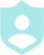###### Example QuestionsWrite the slope-intercept form of the equation of each line.Write the slope-intercept form of the equation of each line.
##### Description :##### Difficulty :GRID 6 Categories 36 QuestionsPrintShareAdd
Questions
Number of categoriesQuestions per categoryCategory 1
GraphsCategory 2
Slope InterceptCategory 3
Standard FormCategory 4
Point SlopeCategory 5
Parallel and PerpendicularCategory 6
Two PointsLayout Preview
 Question Q. Write the slope-intercept form of the equation of each line. A. y = 3x + 4 Question Q. Write the slope-intercept form of the equation of each line given the slope and y-intercept. Slope = 2, y-intercept = -3 A. y = 2x - 3 Question Q. Write the slope-intercept form of the equation of each line. x - 5 y = 5 A. MULTIMEDIA Question Q. Write the slope-intercept form of the equation of the line through the given point with the given slope. through: (3, 5), slope = 1 A. y = x + 2 Question Q. Write the slope-intercept form of the equation of the line described. through: (-4, 3), parallel to y = 2x - 4 A. y = 2x + 11 Question Q. Write the slope-intercept form of the equation of the line through the given points. through: (0, 3) and (5, 2) A. MULTIMEDIA Question Q. Write the slope-intercept form of the equation of each line. A. MULTIMEDIA Question Q. Write the slope-intercept form of the equation of each line given the slope and y-intercept. Slope = -1, y-intercept = -5 A. y = -x - 5 Question Q. Write the slope-intercept form of the equation of each line. 6x + 5 y = -20 A. MULTIMEDIA Question Q. Write the slope-intercept form of the equation of the line through the given point with the given slope. A. MULTIMEDIA Question Q. Write the slope-intercept form of the equation of the line described. A. MULTIMEDIA Question Q. Write the slope-intercept form of the equation of the line through the given points. through: (1, 4) and (0, -5) A. y = 9x - 5 Question Q. Write the slope-intercept form of the equation of each line. A. y = 2x - 3 Question Q. Write the slope-intercept form of the equation of each line given the slope and y-intercept. A. MULTIMEDIA Question Q. Write the slope-intercept form of the equation of each line. 2x - y = -1 A. y = 2x + 1 Question Q. Write the slope-intercept form of the equation of the line through the given point with the given slope. A. MULTIMEDIA Question Q. Write the slope-intercept form of the equation of the line described. through: (-2, -4), parallel to y = x - 3 A. y = x - 2 Question Q. Write the slope-intercept form of the equation of the line through the given points. through: (-2, 1) and (-4, -1) A. y = x + 3 Question Q. Write the slope-intercept form of the equation of each line. A. MULTIMEDIA Question Q. Write the slope-intercept form of the equation of each line given the slope and y-intercept. A. MULTIMEDIA Question Q. Write the slope-intercept form of the equation of each line. x = 4 A. x = 4 Question Q. Write the slope-intercept form of the equation of the line through the given point with the given slope. through: (-2, 1), slope = -2 A. y = -2x - 3 Question Q. Write the slope-intercept form of the equation of the line described. through: (2, -2), perp. to y = 2x + 1 A. MULTIMEDIA Question Q. Write the slope-intercept form of the equation of the line through the given points. through: (-4, 3) and (2, -4) A. MULTIMEDIA Question Q. Write the slope-intercept form of the equation of each line. A. y = 3x - 2 Question Q. Write the slope-intercept form of the equation of each line given the slope and y-intercept. A. MULTIMEDIA Question Q. Write the slope-intercept form of the equation of each line. 3x - 4 y = 28 A. MULTIMEDIA Question Q. Write the slope-intercept form of the equation of the line through the given point with the given slope. A. MULTIMEDIA Question Q. Write the slope-intercept form of the equation of the line described. A. MULTIMEDIA Question Q. Write the slope-intercept form of the equation of the line through the given points. through: (0, 2) and (-3, 0) A. MULTIMEDIA Question Q. Write the slope-intercept form of the equation of each line. A. MULTIMEDIA Question Q. Write the slope-intercept form of the equation of each line given the slope and y-intercept. Slope = -3, y-intercept = -3 A. y = -3x - 3 Question Q. Write the slope-intercept form of the equation of each line. 3x + y = 1 A. y = -3x + 1 Question Q. Write the slope-intercept form of the equation of the line through the given point with the given slope. through: (5, -5), slope = undefined A. x = 5 Question Q. Write the slope-intercept form of the equation of the line described. A. MULTIMEDIA Question Q. Write the slope-intercept form of the equation of the line through the given points. through: (0, 1) and (-5, -4) A. y = x + 1
Colors :
Font color
Button color
Background color
Logo:Background Image:Theme Song: